# Sketch the function with its (a) odd periodic extension and (b) even then find the Fourier...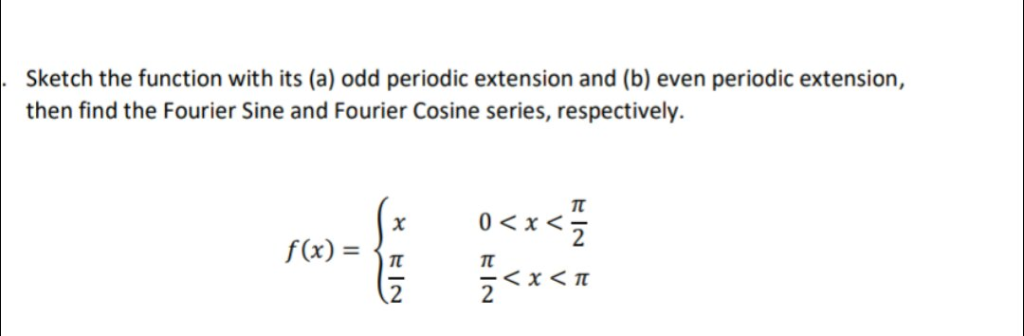Sketch the function with its (a) odd periodic extension and (b) even then find the Fourier Sine and Fourier Cosine series, respectively. periodic extension, 0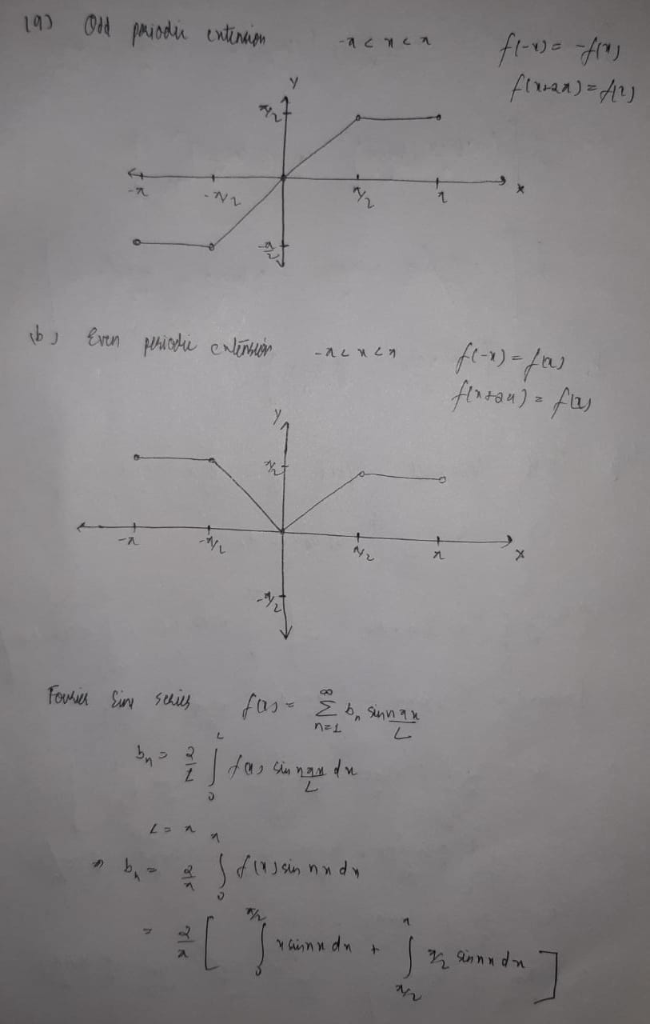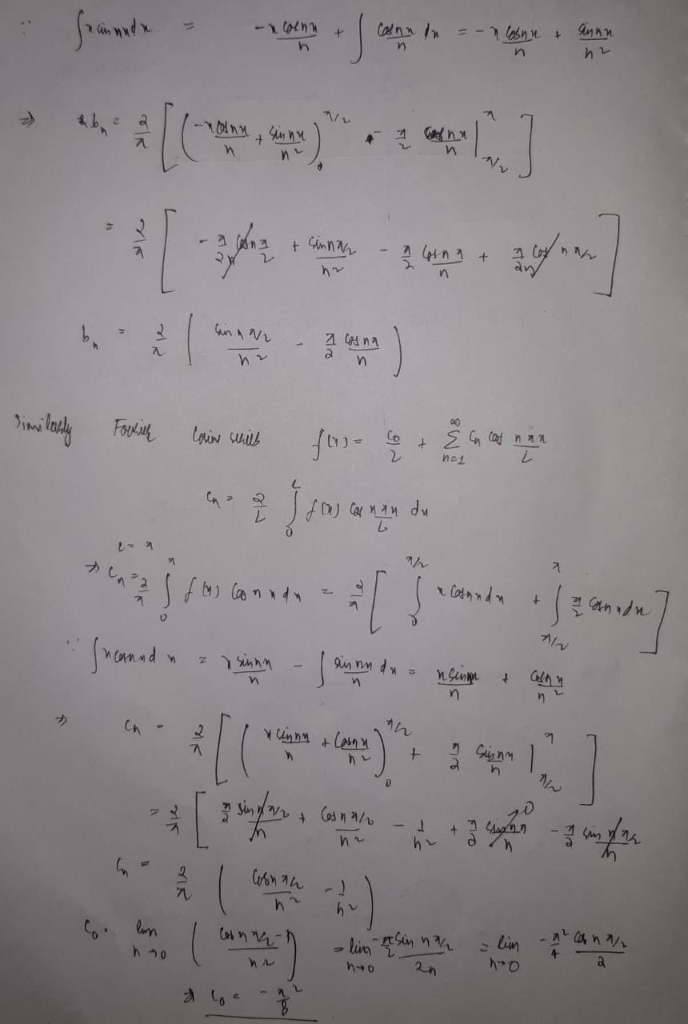##### Add Answer of: Sketch the function with its (a) odd periodic extension and (b) even then find the Fourier...
Similar Homework Help Questions
• ### Consider the function 0<x<π/2. z, f(x) = (a) Sketch the odd and even periodic extension of f(x) for-3π 〈 x 〈 3π...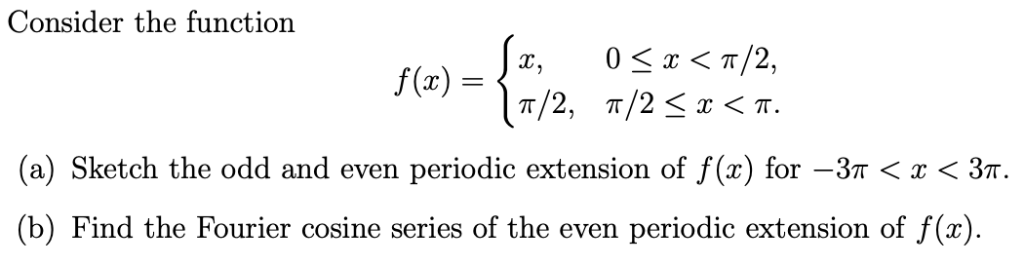Consider the function 0<x<π/2. z, f(x) = (a) Sketch the odd and even periodic extension of f(x) for-3π 〈 x 〈 3π. (b) Find the Fourier cosine series of the even periodic extension of f(x) Consider the function 0

• ### 5. (a) (6) Carefully sketch the odd periodic extension, of period 2m, of the function f(x)1, 0 < x < π. (Only sketch over the interval z E [-2π, 2π). (b) (10) Find the Fourier sine series o...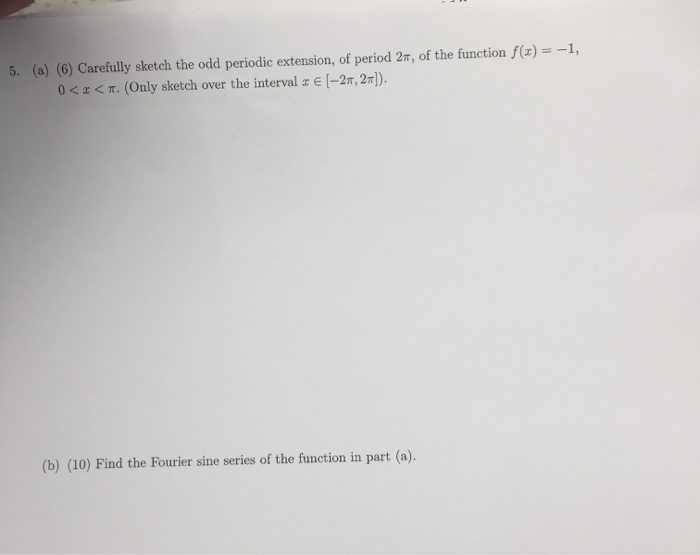5. (a) (6) Carefully sketch the odd periodic extension, of period 2m, of the function f(x)1, 0 < x < π. (Only sketch over the interval z E [-2π, 2π). (b) (10) Find the Fourier sine series of the function in part (a) 5. (a) (6) Carefully sketch the odd periodic extension, of period 2m, of the function f(x)1, 0

• ### 1. Consider the function defined by 1- x2, 0< |x| < 1, f(x) 0, and f(r) f(x+4) (a) Sketch the graph of f(x) on th...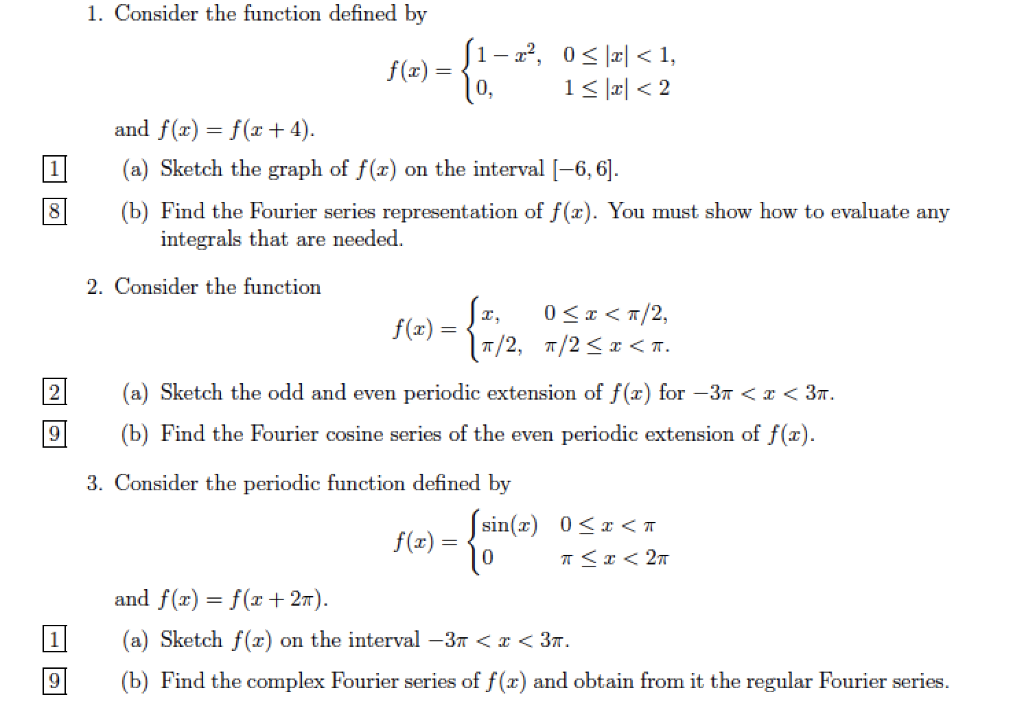1. Consider the function defined by 1- x2, 0< |x| < 1, f(x) 0, and f(r) f(x+4) (a) Sketch the graph of f(x) on the interval -6, 6] (b) Find the Fourier series representation of f(x). You must show how to evaluate any integrals that are needed 2. Consider the function 0 T/2, T/2, T/2 < T. f(x)= (a) Sketch the odd and even periodic extension of f(x) for -3r < x < 3m. (b) Find the Fourier cosine series...

• ### cosine and sine fourier series, Half Range Expansions

Find the half-range expansions for: f (x) = 1- 2x (0 < x <1/2)i.e.(1) Find the Fourier cosine series (even extension), and(2) Find the Fourier sine series (odd extension).

• ### 1. Cousider the followving periodic function a) Determine whether the following function is odd, even or...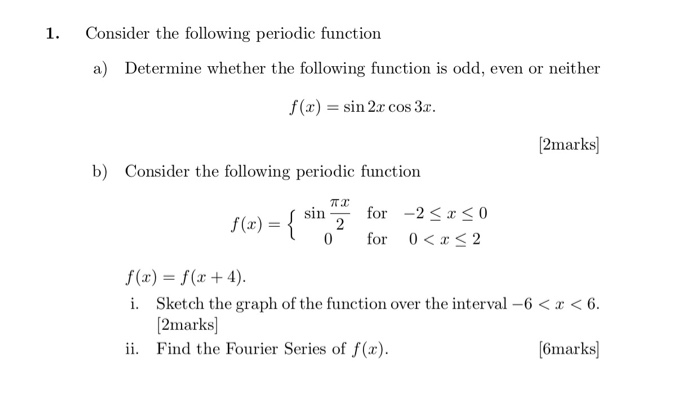1. Cousider the followving periodic function a) Determine whether the following function is odd, even or neither f(x) = sin 2x cos 3a. 2marks] Consider the following periodic function b) ㄫㄨ for -2 < x < 0 for 0< S 2 f(x) = { sin 0 f(x) = f(x + 4). i. Sketch the graph of the function over the interval-6< r <6. 2marks] Find the Fourier Series of f(x). (6marks ii.

• ### 0.2 Find the Fourier seris for (periodic extension of) 1, t e [0,2): f(t) = (-1, t E [2,4). Determine the sum of this series. 2. Find the Fourier series for (periodic extension of) t 1, te[0, 2):...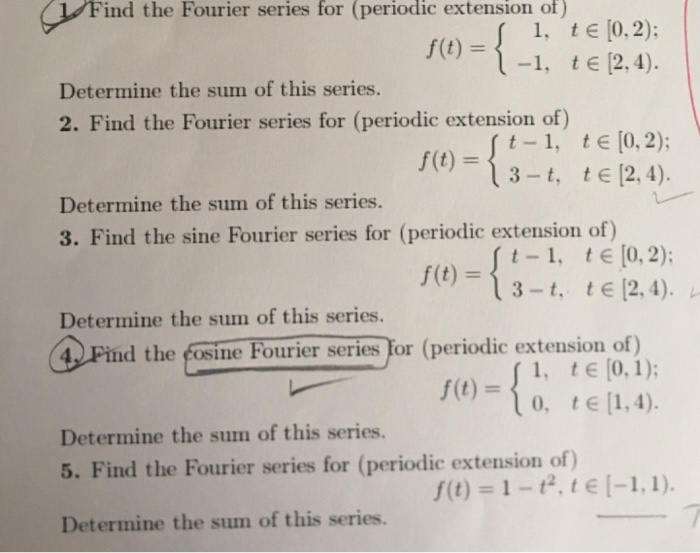0.2 Find the Fourier seris for (periodic extension of) 1, t e [0,2): f(t) = (-1, t E [2,4). Determine the sum of this series. 2. Find the Fourier series for (periodic extension of) t 1, te[0, 2): 3-t, te[2, 4) Determine the sum of this series. 3. Find the sine Fourier series for (periodic extension of) t -1, t[o,2) , (t)- Determine the sum of this series. 4 Pind the Fosine Fourier series for (periodic extension of) 1, tE...

• ### x2 when x E [0,1]. 1. (Total marks 12) Suppose f(x) (a) Sketch the periodic odd...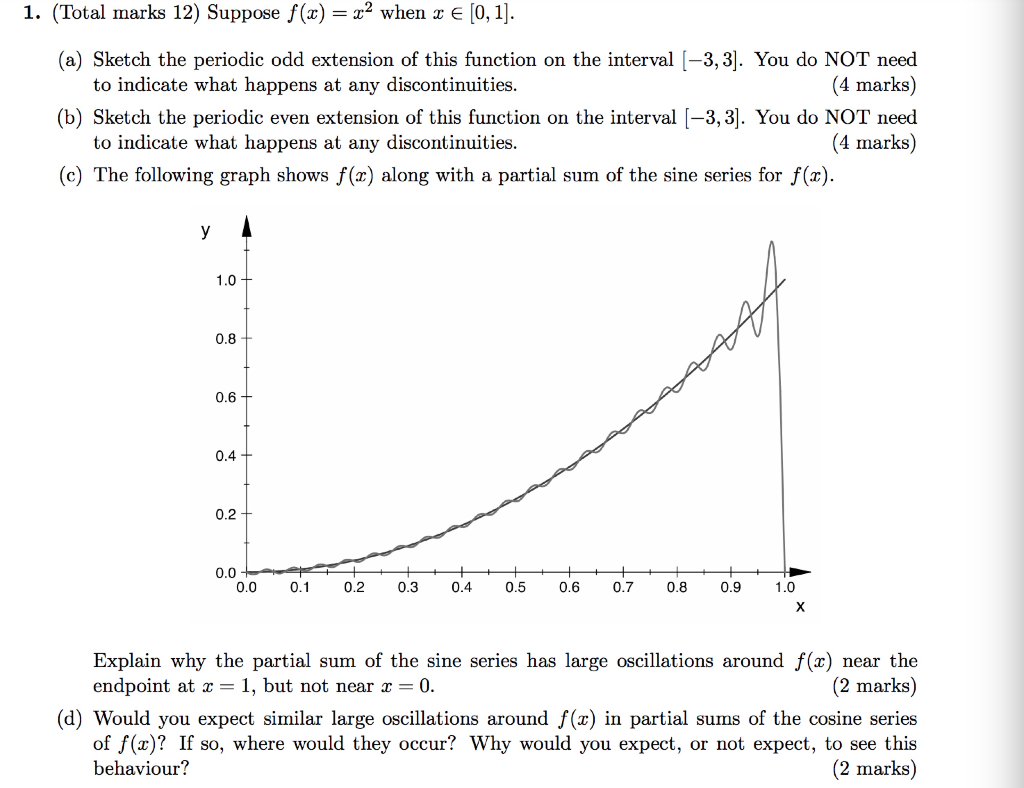x2 when x E [0,1]. 1. (Total marks 12) Suppose f(x) (a) Sketch the periodic odd extension of this function on the interval [-3,31. You do NOT need to indicate what happens at any discontinuities. (4 marks) (b) Sketch the periodic even extension of this function on the interval -3,31. You do NOT need to indicate what happens at any discontinuities (4 marks) (c) The following graph shows f (x) along with a partial sum of the sine series for...

• ### 3. Consider the function defined by f(x) = 1, 0 < r< a, | 0, a<...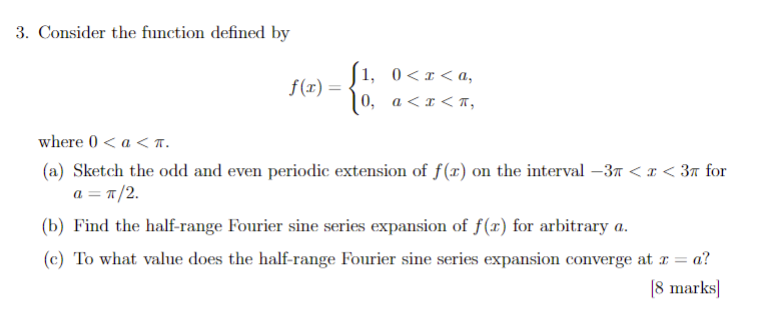3. Consider the function defined by f(x) = 1, 0 < r< a, | 0, a< x < T, where 0a < T (a) Sketch the odd and even periodic extension of f (x) on the interval -3n < x < 3« for aT/2 (b) Find the half-range Fourier sine series expansion of f(x) for arbitrary a. (e) To what value does the half-range Fourier sine series expansion converge at r a? [8 marks 3. Consider the function defined by...

• ### There are 3 questions on this assignment. The marks awarded for each part are indi- cated in boxes. 1. Consider the fun...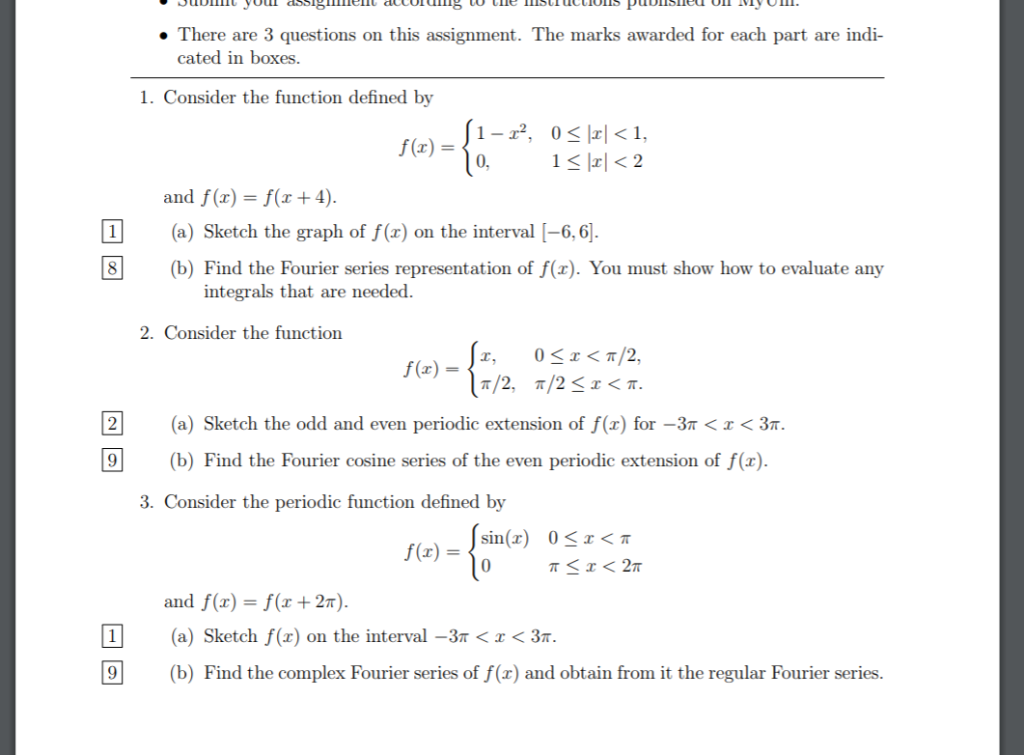There are 3 questions on this assignment. The marks awarded for each part are indi- cated in boxes. 1. Consider the function defined by f(x) = 0 and f(x)-f(x +4) 1 (a) Sketch the graph of f(x) on the interval -6,6 (b) Find the Fourier series representation of f(z). You must show how to evaluate any integrals that are needed 2. Consider the function f(x) (a) Sketch the odd and even periodic extension of f(x) for-3< x < 3m (b)...

• ### Fourier Series for Odd Functions Recall that if f is an odd function, f(-x)f(x). An odd Fourier series has only the...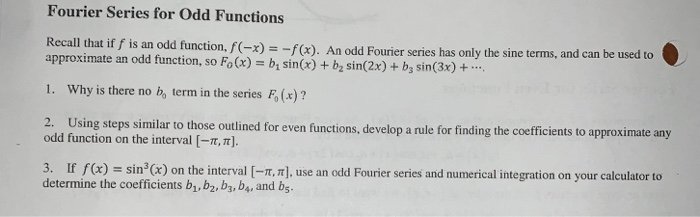Fourier Series for Odd Functions Recall that if f is an odd function, f(-x)f(x). An odd Fourier series has only the sine terms, and can be approximate an odd function, so Fo(x) b sinx)+b2 sin(2x)+ b, sin(3x)+. Why is there no b, term in the series F, (x)? 1. 2. Using steps similar to those outlined for even functions, develop a rule for finding the coefficients to approximate any odd function on the interval [-π, π]. 3. If f (x)sin...

Need Online Homework Help?